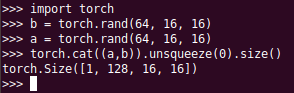# How to stack 2 tensors

Hi everyone;
I’m trying to stuck 2 tensors A.shape=(64,16,16) and B.shape=(64,16,16) in a tensor of shape C.shape=(1,128,16,16) and non of functions i’ve tried work where
torch.stack => C.shape=(2,64,16,16) and torch.cat => C.shape=(128,16,16)
can enyones help me

torch.cat and then you unsqueeze(0) the result1 Like